# Field (mathematics)

A field is a commutative ring which contains a non-zero multiplicative identity and all non-zero elements have multiplicative inverses. Loosely, a field is a collection of entities with well-behaved and compatible addition and multiplication operations. A few examples serve to illustrate this point.

## Examples

1. The rational numbers, with operations the usual addition and multiplication.
2. The real numbers, with operations the usual addition and multiplication.
3. The complex numbers, with operations the usual addition and multiplication.
4. The integers modulo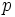(denoted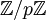), whereis prime. Here the operations are addition and multiplication modulo. Observe that ifis not prime, thenis not a field. For example, the element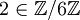has no multiplicative inverse modulo 6! In this case,has only the structure of a ring.
5. The field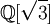of real numbers of the form, where bothandare rational.
6. Finite fields: for each prime numberand positive integer, there is a unique (up to isomorphism) finite field of cardinality is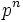. This field is of characteristic.
7. The set of meromorphic functions on a complex manifold, with pointwise addition and multiplication. For example, the set of meromorphic functions onor the unit disk.
8. The p-adic fieldsand, which play a prominent role in number theory.

The characteristic of a field must be either 0 or a prime number p. A field of characteristic 0 is necessarily infinite.

Fields play an important role in nearly every area of mathematics, and are one of the most basic objects studied by algebra. The study of the relationships between different fields, and in particular subfields of a given field, leads to the study of Galois theory, and makes possible the proof of Abel's theorem and was one of the motivations for the early study of fields and abstract algebra more generally.

## Axioms

Technically, a field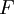is a set of elements endowed with two binary operations,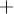and(with properties analogous to addition and multiplication, respectively), which obey the following axioms:

1. Closure: If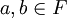then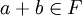.
2. Associativity: For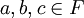,.
3. Commutativity: For.
4. Identity: There exists an elementsuch thatfor all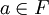.
5. Inverse: For allthere exists an elementsuch that.
• Multiplication axioms
1. Closure: Ifthen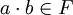.
2. Associativity: For,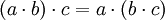.
3. Commutativity: For,.
4. Identity: There exists an elementsuch that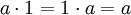for all.
5. Inverse: Ifandthen there exists an element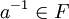such that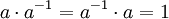.
• Distributivity: For,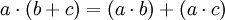.

Sometimes the condition 0 ≠ 1 is also included.